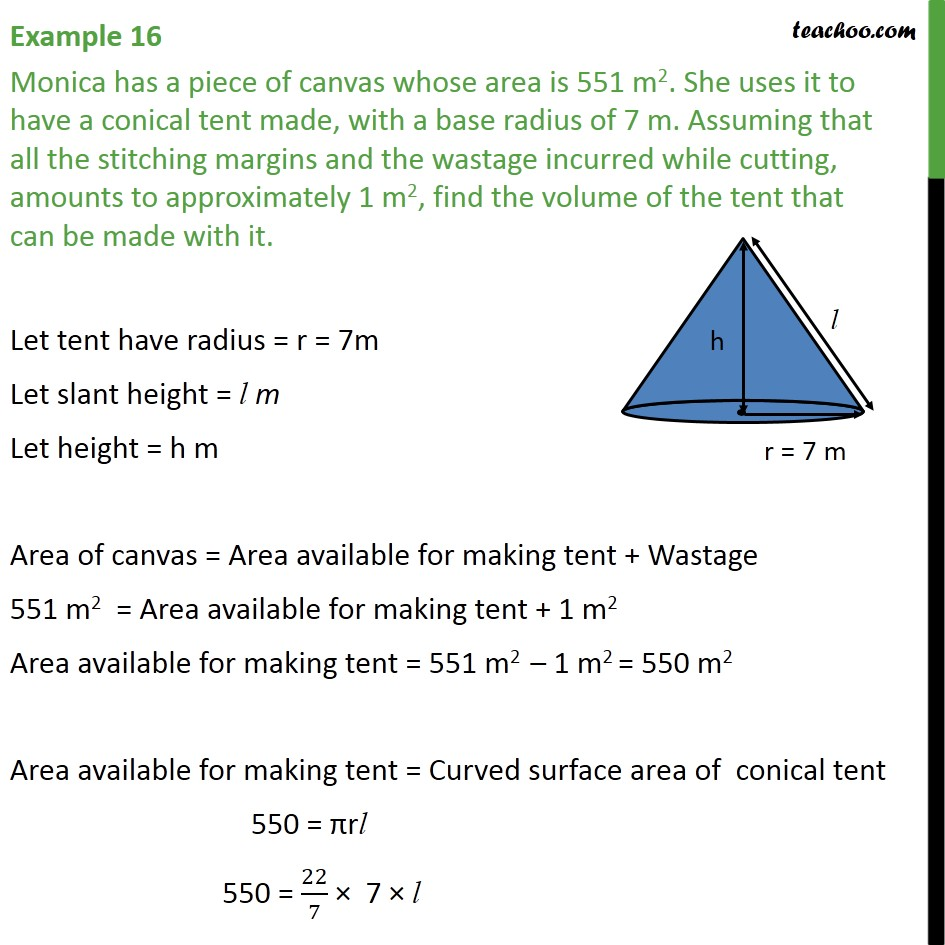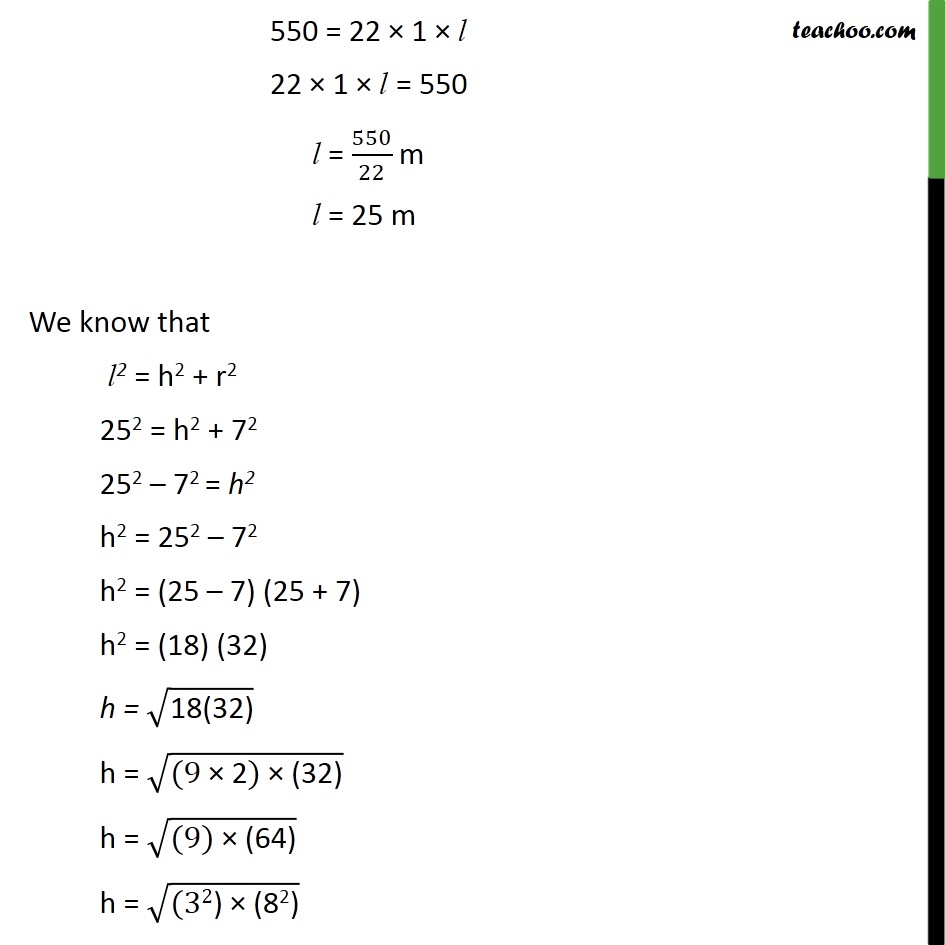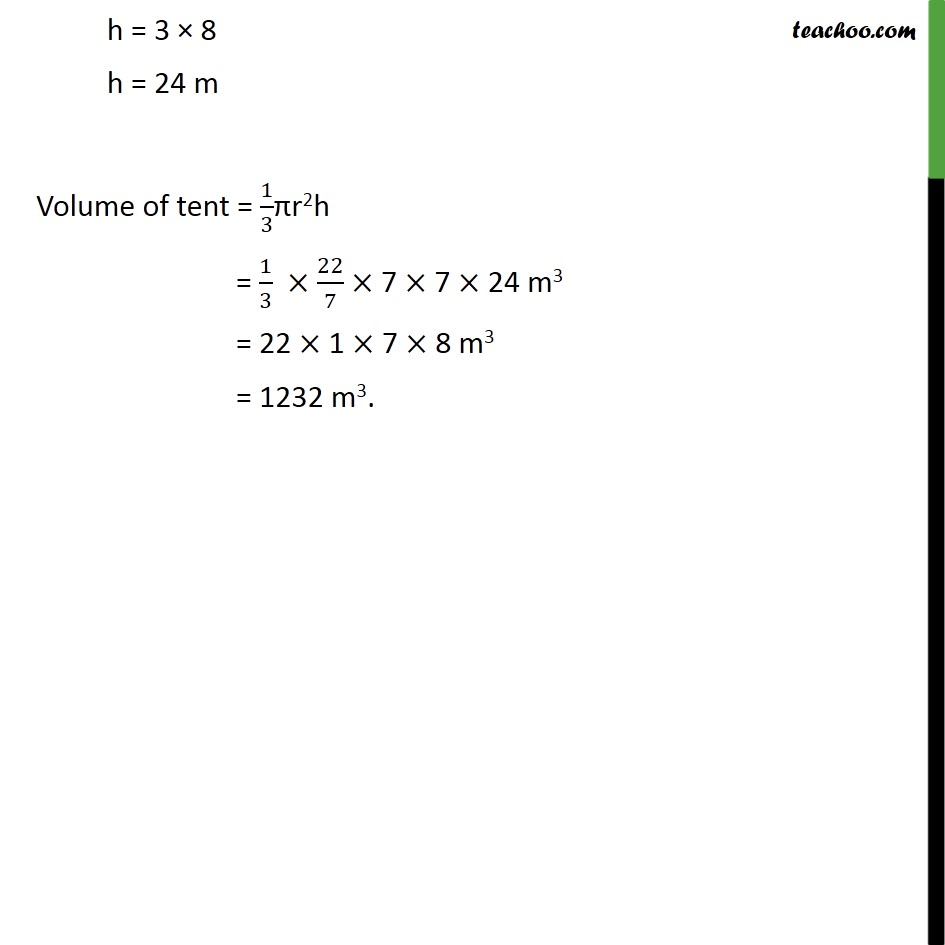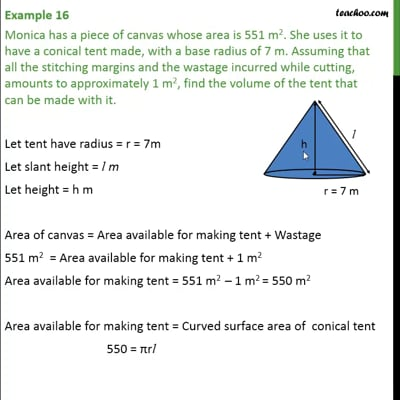Examples

Chapter 13 Class 9 Surface Areas and Volumes
Serial order wiseThis video is only available for Teachoo black users

Maths Crash Course - Live lectures + all videos + Real time Doubt solving!

### Transcript

Example 16 Monica has a piece of canvas whose area is 551 m2. She uses it to have a conical tent made, with a base radius of 7 m. Assuming that all the stitching margins and the wastage incurred while cutting, amounts to approximately 1 m2, find the volume of the tent that can be made with it. Let tent have radius = r = 7m Let slant height = l m Let height = h m Area of canvas = Area available for making tent + Wastage 551 m2 = Area available for making tent + 1 m2 Area available for making tent = 551 m2 – 1 m2 = 550 m2 Area available for making tent = Curved surface area of conical tent 550 = πrl 550 = 22/7 × 7 × l 550 = 22 × 1 × l 22 × 1 × l = 550 l = 550/22 m l = 25 m We know that l2 = h2 + r2 252 = h2 + 72 252 – 72 = h2 h2 = 252 – 72 h2 = (25 – 7) (25 + 7) h2 = (18) (32) h = √("18(32)" ) h = √("(9 × 2) × (32)" ) h = √("(9) × (64)" ) h = √("(32) × (82)" ) h = 3 × 8 h = 24 m Volume of tent = 1/3πr2h = 1/3 × 22/7 × 7 × 7 × 24 m3 = 22 × 1 × 7 × 8 m3 = 1232 m3.